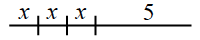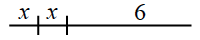### Home > CC1 > Chapter 4 > Lesson 4.1.1 > Problem4-8

4-8.

Now Croakie can do a super high jump!

The first time he performed his new super-high-jump routine, he did three super-high jumps and then hopped five feet. The second time, he did only two super-high jumps and then hopped six feet. Both times, he covered the same distance. His attempts are shown in the diagram at right.

First sequenceSecond sequence1. How far does Croakie travel in one super-high jump? Explain or show how you know.

In Croakie's first attempt he crossed the tightrope like this: $x + x + x + 5 = \text{length of the tightrope}$

In Croakie's second attempt he crossed the tightrope like this: $x + x + 6 = \text{length of the tightrope}$

Because both equations are equal to the length of a tightrope, you can make them equal to each other,
like this: $x + x + x + 5 = x + x + 6$

$1$ foot. Make sure you know how to get this answer.

2. How long is his whole super-high-jump routine? How can you tell?

• Since you figured out that Croakie's super-high jump ($x$) equals $1$ foot, you can simply substitute $1$ for $x$ into either of his routines.